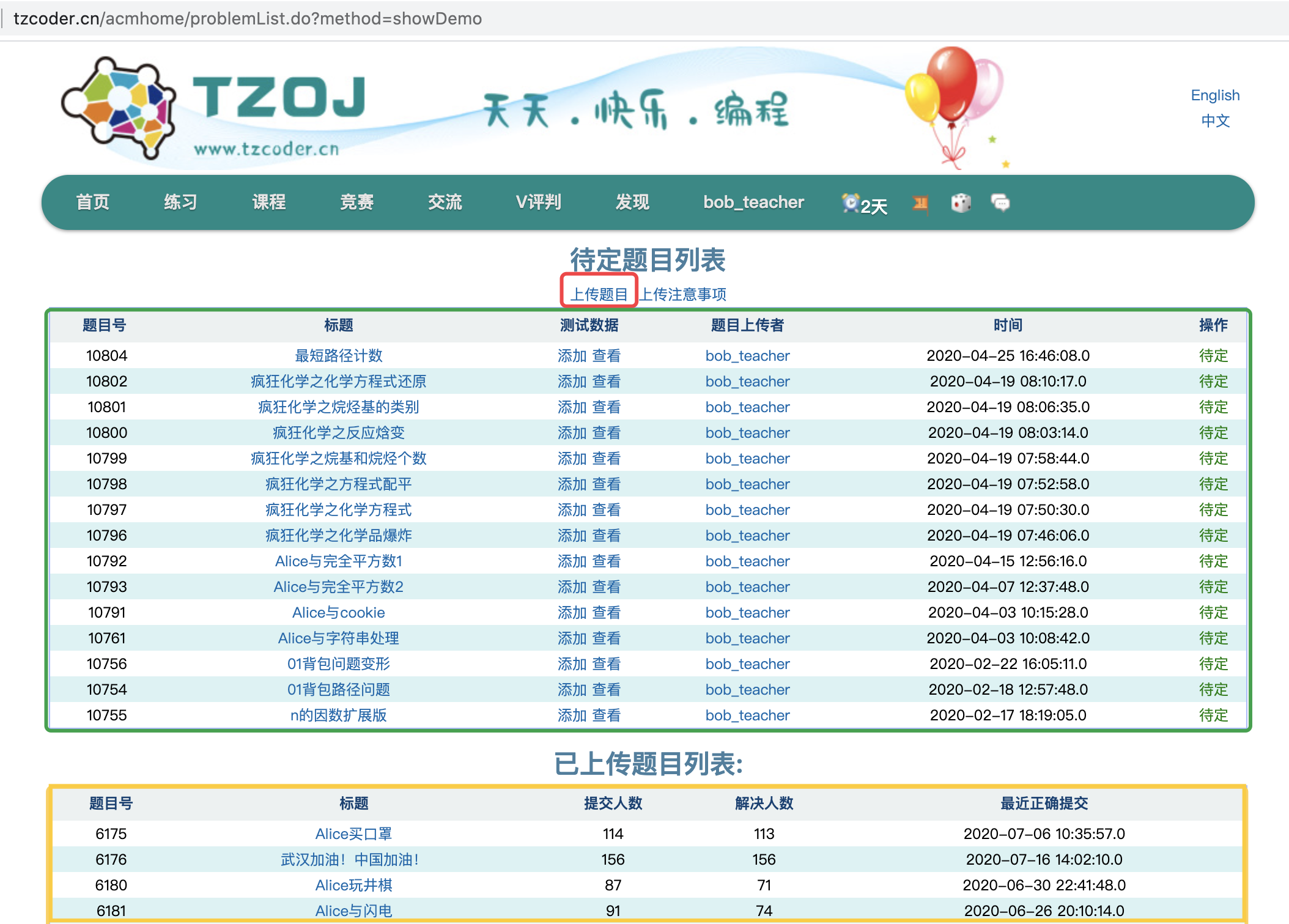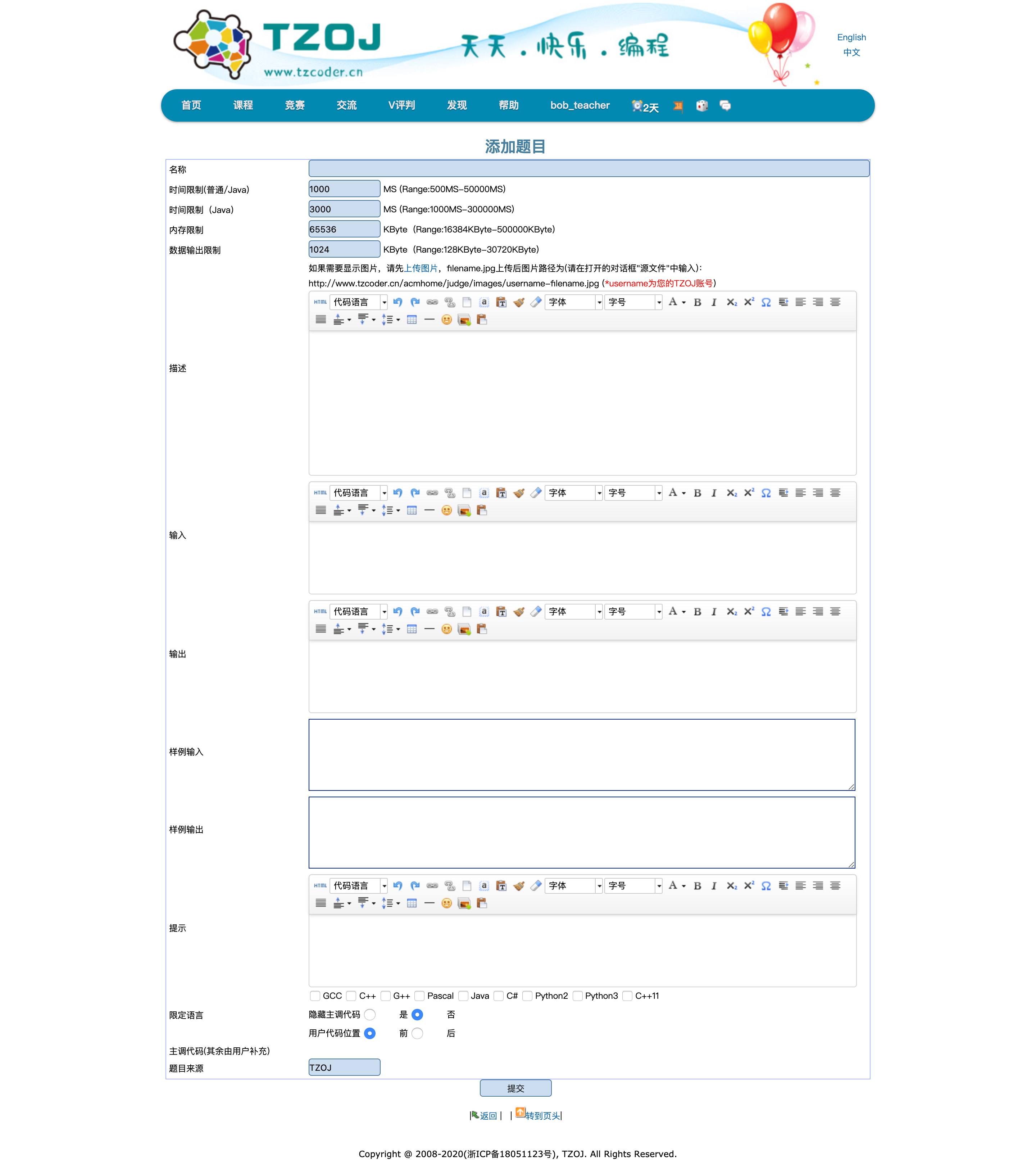# 一、确定题目并撰写题面可以看到如下界面# 二、制造数据及提交标程

C++ 库有一个名为 rand() 的函数，每次调用该函数都将返回一个非负整数。

#include <bits/stdc++.h>
using namespace std;

int main()
{
//循环随机100次
for(int i=0;i<100;i++)
{
int x=rand();
cout<<x<<"\n";
}
return 0;
}


rand函数返回的数字其实是伪随机数。它们虽然具有随机数的表现和属性，但实际上并不是随机的。它们实际上是用算法生成的。各种各样的伪随机数算法可以了解一下。

#include <bits/stdc++.h>
using namespace std;

int main()
{
srand(time(NULL));
//循环随机100次
for(int i=0;i<100;i++)
{
int x=rand();
cout<<x<<"\n";
}
return 0;
}


#include <bits/stdc++.h>
using namespace std;
int main()
{
//把当前时间作为随机数种子，没有这一步你的随机是假的
srand(time(NULL));
//可以生成[1000,10000)的一个整数
int n=0;
while(n<=1000)
{
n=rand()%10000;
}
//显然上面这个效率很差，特别是区间很小的话，你可以随机一个[0,9000）的数+1000就好了
n=rand()%9000+1000;
cout<<n<<"\n";
}


#include <bits/stdc++.h>
using namespace std;
int main()
{
srand(time(NULL));
int a, n = 20;
for (int i = 0; i < n; i++)
a[i] = i + 1;
random_shuffle(a, a + n);
for (int i = 0; i < n; i++)
cout << a[i] << "\n";
return 0;
}


#include<bits/stdc++.h>
using namespace std;
int main()
{
//所有会被存储到当前文件夹下的data1.in文件下
freopen("data1.in","w",stdout);
srand(time(NULL));
int T=50;
cout<<T<<"\n";
while(T)
{
//m和n都是[1,9],rand%9是[0,8],+1就是了
int n=rand()%9+1,m=rand()%9+1;
//flag用来标记只出现一个s
int flag=0;
string a;
for(int i=0;i<n;i++)
{
for(int j=0;j<m;j++)
{
//x的值为[0,2]
int x=rand()%3;
//随机出2就是s，但是只能有一个
if(x==1)
a[i]+="d";
else if(x==2&&flag==0)
a[i]+="s",flag=1;
else
a[i]+=".";
}
}
//用来检验是否合法
int flag1=0,flag2=0;
for(int i=0;i<n;i++)
{
for(int j=0;j<m;j++)
{
if(a[i][j]=='s')
{
flag1++;
}
if(a[i][j]=='d')
{
flag2++;
}
}
}
//'s'只能有一个，d存在
if(flag1==1&&flag2)
{
//输出当前的n和m以及当前构造的数据
cout<<n<<" "<<m<<"\n";
for(int i=0;i<n;i++)
a[i][m]=0,cout<<a[i]<<"\n";
//成功了就可以少构造一组了
T--;
}
}
return 0;
}


    freopen("data1.in","r",stdin);
freopen("data1.out","w",stdout);


TZOJ还支持Special Judge，即有些题目会显示红色的Special Judge提示，表示选手的输出不一定要和你的相同，如果需要这个功能，请联系管理员。

# 三、管理员通过

posted @ 2020-04-22 09:25  暴力都不会的蒟蒻  阅读(147)  评论(0编辑  收藏